Parallel and Perpendicular Lines
Angles
Transformations
Congruence Statements
Proofs
Anything Goes!
100

Define parallel lines AND perpendicular lines.

Parallel: Lie in the same plane and never intersect.

Perpendicular: Intersect to form RIGHT ANGLES.

100

Congruent means _______.

Supplementary means _______.

Equal

100

Find the measure of <A.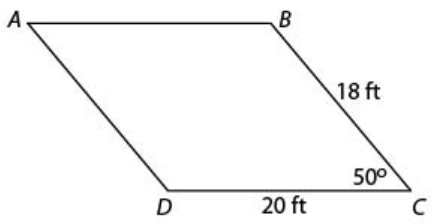<A = 50o

100
List the transformations that are also rigid motions.

What do we know about rigid motions?

Translation, rotation, reflection.

Preserve congruence.

100

What side is congruent to side EG?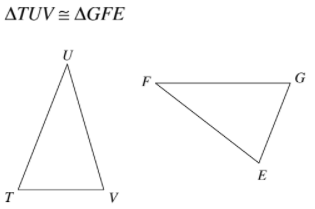Side VT

100

Complete the proof below.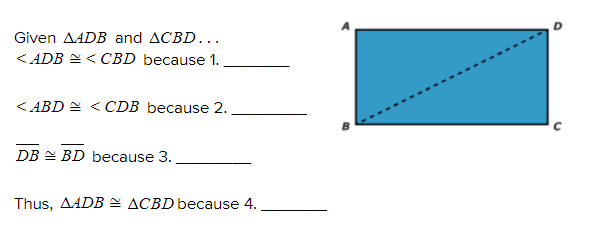1. Alternate interior angles are congruent

2. Alternate interior angles are congruent

3. Reflexive property

4. ASA

100

What is the rotational symmetry of a regular hexagon?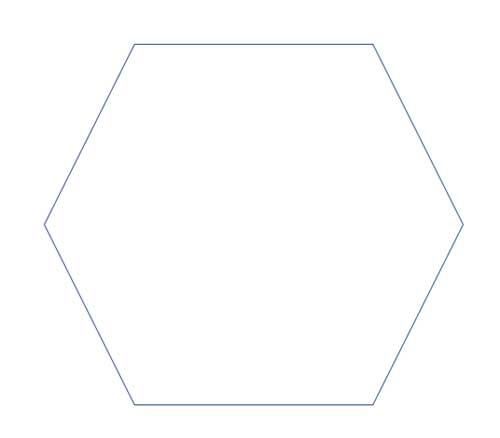360/6=60

200

Parallel lines have slopes that are ___________, while perpendicular lines have slopes that are _________.

Equal; Opposite reciprocals.

200

What angles are congruent to <1?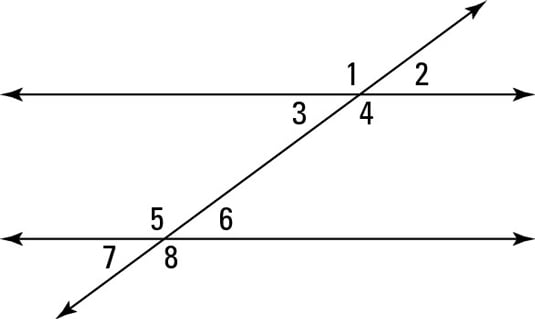<4, <5, <8

200

Find the measure of <D.<D = 130o

200

Translate the point A(-4, -4)  by (x + 3, y + 4).

A'(-1, 0)

200

What angle is congruent to <FED?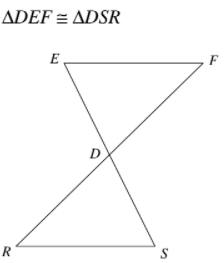<RSD

200

Complete the proof listed below.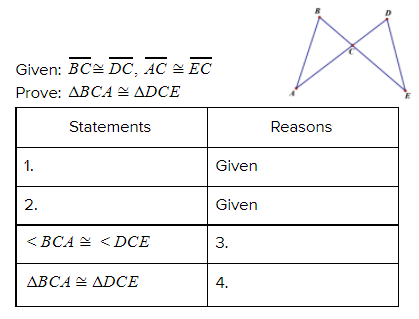1. BC ≅ DC

2. AC ≅ EC

3. Vertical angles are congruent.

4. SAS

200

Solve the equation.

4x-3=65

x=17

300

Identify the slope and y-intercept of the following line:

y=-6x-2

m=-6;b=-2

300

Identify the angle relationship.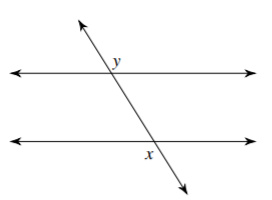Alternate exterior angles

300

Solve for x.x=0

300

If  B(3,1) and B'(-4, 5), what is the translation vector?

< -7, 4>

300

Write the six corresponding congruent parts of the triangles shown below.DE≅DS; EF≅SR; DF≅DR; <DEF≅<DSR; <EFD≅<SRD; <FDE≅RDE

300

Complete the proof listed below.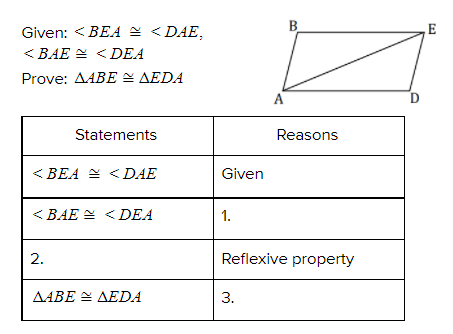1. Given

2. AE ≅ EA

3. ASA

300

8/7 +4/3=

52/21

400

Write the equation of a line that passes through the point (-1,3) and is parallel to the line below.

y=-6x-2

Slope of original line: -6

Slope of new line: -6

Plug -6 and the points (-1, 3) into y=mx+b

y=-6x-3

400

Find the value of the missing angle.113o

400

Find the value of x.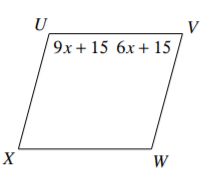x = 10

400

Reflect the triangle M(2,0), E(3,1), L(-1,2) about the line x= -3.

M'(-8,0); E'(-9,1); L'(-5,2)

400

Label the corresponding congruent sides and angles on the triangles below.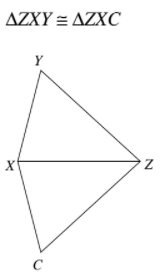400

Complete the proof below.1. Given

4. Alternate interior angles are congruent

5. BD ≅ DB

6. AAS

400

4/5+1/2+5/4=

51/20

500

Write the equation of a line that passes through the point (12,1) and is perpendicular to the line below.

y=-6x-2

Slope of original line: -6

Slope of new line: 1/6

Plug 1/6 and the points (12, 1) into y=mx+b

y=1/6x-1

500

Find the value of x.x=-9

500

What property tells us that side AC is congruent to itself?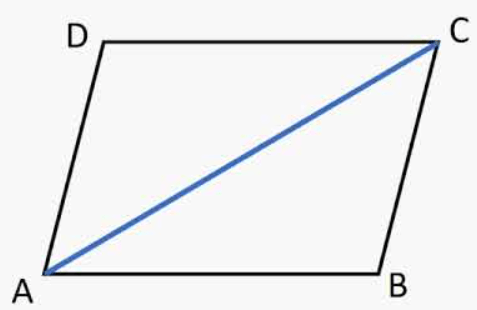Reflexive property

500

Dilate Z(-1, 0), G(0, 2), E(1, 3), W(-1, -1.5) by a scale factor of 2.5.

Z'(-2.5, 0); G'(0, 5); E'(2.5, 7.5); W'(-2.5, -3.75)

500

Label the corresponding congruent sides and angles on the triangles below.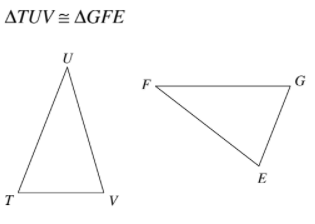500

Complete the proof below.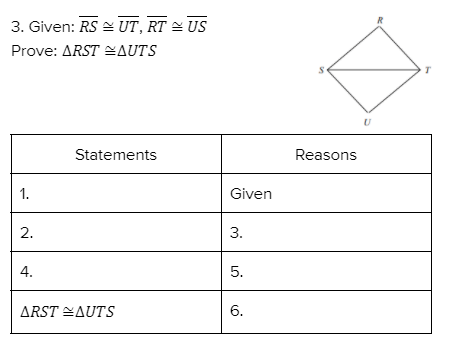1. RS ≅ UT

2. RT ≅ US

3. Given

4. ST ≅ TS

5. Reflexive property

6. SSS

500

How many squares are there?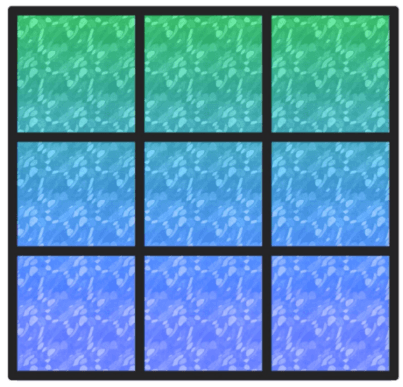14 squares

600

Is the line passing through the points (−4,5) and (1,10) parallel to the line passing through the points (−18,−6) and (−8,4)?

m=1

m=1

Since the slopes of the lines are the same, the lines are parallel.

600

Find the value of x.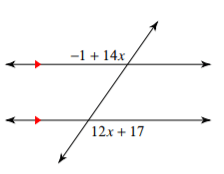x=9

600

Name one characteristic of ALL parallelograms.

- Opposite sides that are parallel.

- Opposite sides are congruent.

- Opposite angles are congruent.

- Consecutive angles are supplementary.

600

Rotate the following points 180° clockwise about the origin: L(1, 3), Z(5, 5), F(4, 2).

L'(−1, −3); Z'(−5, −5); F'(−4, −2)

600

Label the corresponding congruent sides and angles on the triangles below.600

Complete the proof below.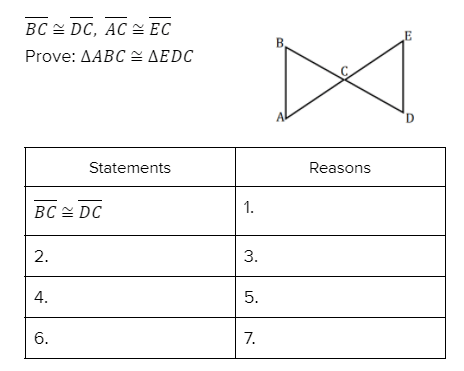1. Given

2. AC ≅ EC

3. Given

4. <BCA ≅ DCE

5. Vertical angles are congruent

6. Triangle ABC ≅ Triangle EDC

7. SAS

600

There are five people in a meeting. Every person wants to shake the hands of everyone else in the room once. How many handshakes will occur?

10 handshakes

Click to zoom